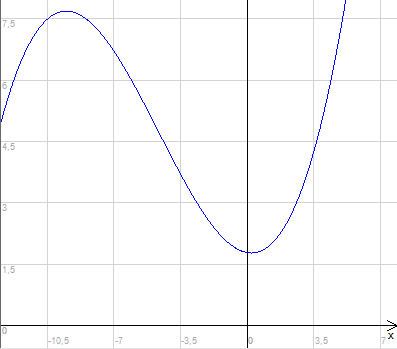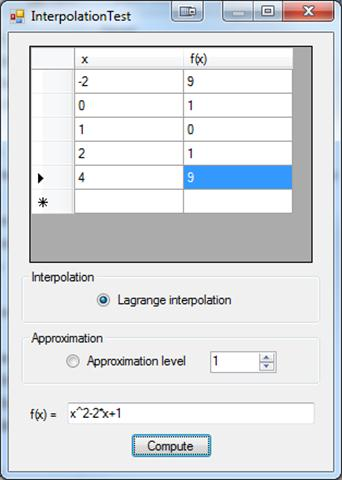### Features And Algorithms

Interpolation uses my own algorithm for calculating Lagrange coefficients. Approximation uses standard formula for building simultaneous equations and solves them using Gaussian elimination.

### Examples and usage

You can use this library to find function formula that goes through all given points. For example having {-2, 4}, {0, 0}, {1, 1} and {2, 4} we will receive f(x)=x^2 as result.

`// VARIABLESList<PointD> points = new List<PointD>();points.Add(new PointD(-2, 4));points.Add(new PointD(0, 0));points.Add(new PointD(1, 1));points.Add(new PointD(2, 4));//ComputingInterpolation interpolation = new Interpolation(points);string result = interpolation.Compute();`

Approximation returns function formula that goes near given points and is a polynomial of requested degree. For example approximation of {-4, 5}, {-2, 3}, {0, 1}, {2, 3}, {5, 5} requesting third degree will give us function as below:

`// VARIABLESint level = 3;List<PointD> points = new List<PointD>();points.Add(new PointD(-4, 5));points.Add(new PointD(-2, 3));points.Add(new PointD(0, 1));points.Add(new PointD(2, 3));points.Add(new PointD(5, 5));//ComputingApproximation approximation = new Approximation(points, level);string result = approximation.Compute();`### Example application